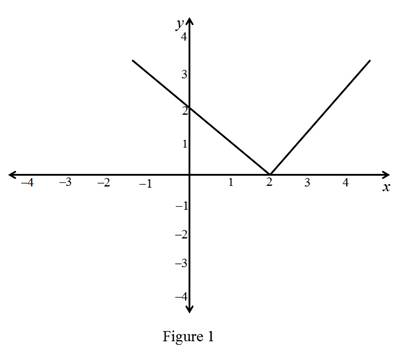# The meaning for f to be differentiable at a.### Single Variable Calculus: Concepts...

4th Edition
James Stewart
Publisher: Cengage Learning
ISBN: 9781337687805### Single Variable Calculus: Concepts...

4th Edition
James Stewart
Publisher: Cengage Learning
ISBN: 9781337687805

#### Solutions

Chapter 2, Problem 14RCC

(a)

To determine

## To describe: The meaning for f to be differentiable at a.

Expert Solution

### Explanation of Solution

If a is appoint in the domain of a function f, then f is said to be differentiable at a if the derivative f(a) exists. This means the graph of f has non-vertical tangent line at the point (a,f(a)). If f is differentiable at a point a then f must be continuous at a.

(b)

To determine

Expert Solution

### Explanation of Solution

All differentiable functions are continuous, but not all the continuous functions are differentiable.

Let a function y=f(x) is differentiable at a point x0 in its domain only if f(x) is continuous at x0.

Function y=f(x) is differentiable at a point x0 is

f(x0)=limΔx0ΔyΔx          =limxx0f(x)f(x0)xx0

Since, x approaches x0 implies xx0, then

f(x)f(x0)=f(x)f(x0)xx0(xx0).

Take limit as x approaches to x0 of both sides

limxx0(f(x)f(x0))=limxx0(f(x)f(x0)xx0(xx0))                              =f(x0)(0)=0

Hence limxx0f(x)=f(x0).

Since there exist limxx0f(x)=f(x0) and x0 is contained in the domain of f, f(x) is continuous at x0.

(c)

To determine

Expert Solution

### Explanation of Solution

Example:

Let the function f(x)=|x2| and  f is continuous but it is not differentiable at 2.

Graph:### Have a homework question?

Subscribe to bartleby learn! Ask subject matter experts 30 homework questions each month. Plus, you’ll have access to millions of step-by-step textbook answers!